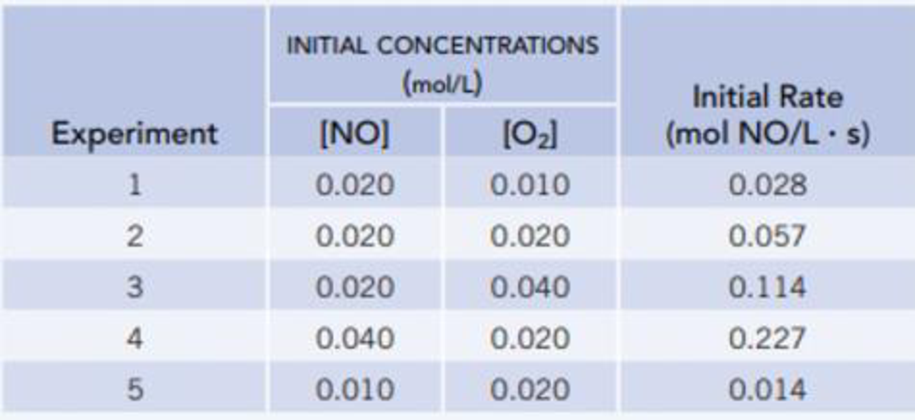# The initial rate (‒ Δ[NO]/ Δ t ] of the reaction of nitrogen monoxide and oxygen NO(g) + ½2O 2 (g) → NO 2 (g) was measured for various initial concentrations of NO and O 2 at 25 °C. Determine the rate equation from these data. What is the value of the rate constant, k , and what are its units?### Chemistry & Chemical Reactivity

9th Edition
John C. Kotz + 3 others
Publisher: Cengage Learning
ISBN: 9781133949640

#### Solutions

Chapter
Section### Chemistry & Chemical Reactivity

9th Edition
John C. Kotz + 3 others
Publisher: Cengage Learning
ISBN: 9781133949640
Chapter 14.3, Problem 1CYU
Textbook Problem
171 views

## The initial rate (‒ Δ[NO]/ Δt] of the reaction of nitrogen monoxide and oxygenNO(g) + ½2O2(g) → NO2(g)was measured for various initial concentrations of NO and O2 at 25 °C. Determine the rate equation from these data. What is the value of the rate constant, k, and what are its units?Interpretation Introduction

Interpretation: The reaction rate and the value of rate constant has to be given.

Concept introduction:

Rate law or rate equation: The relationship between the reactant concentrations and reaction rate is expressed by an equation.

aA + bBxXRate of reaction = k [A]m[B]n

Order of a reaction: The order of a reaction with respect to a particular reactant is the exponent of its concentration term in the rate law expression, and the overall reaction order is the sum of the exponents on all concentration terms.

Rate constant, k: It is a proportionality constant that relates rate and concentration at a given temperature.

### Explanation of Solution

The reaction rate of the chemical reaction is given as,

Reaction Rate = k [NO]m[O2]n,where m, and n are orders of the reactants.Givenreaction: NO(g)+ 1/2 O2(g)®NO2(g)Orderofthereaction:_*Comparingfirsttwoexperiments1and2,rate1=[NO]1m[O2]1n, rate1 = 0.028 mol/L.hrate2 = k [NO]2m[O2]2n, rate 2 = 0.057 mol/L.hrate2rate1=k [NO]2m[O2]2nk [NO]1m[O2]1n0.057mol/L.h0.028mol/L.h=(0.020)m(0.020)n(0.020)m(0.010)n 2.0 = (2)nn = 1Comparinglasttwoexperiments2and4,rate2 =[NO]2m[O2]2n,rate 2 = 0.057 mol/L.hrate4 = k [NO]4m[O2]4n,rate 4 = 0.227 mol/L.hrate 4rate2 =k [NO]4m[O2]4nk [NO]2m[O2]2n0

### Still sussing out bartleby?

Check out a sample textbook solution.

See a sample solution

#### The Solution to Your Study Problems

Bartleby provides explanations to thousands of textbook problems written by our experts, many with advanced degrees!

Get Started

Find more solutions based on key concepts
American restaurant portions are stable and consistent; use them as a guide to choosing portion sizes. T F

Nutrition: Concepts and Controversies - Standalone book (MindTap Course List)

Two cities in Germany are located 25km apart. What is the distance in miles?

Chemistry for Today: General, Organic, and Biochemistry

An aluminum rod is clamped one-fourth of the way along its length and set into longitudinal vibration by a vari...

Physics for Scientists and Engineers, Technology Update (No access codes included)